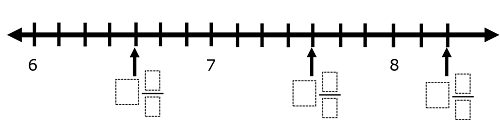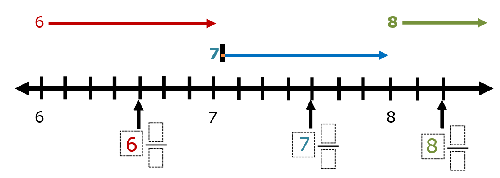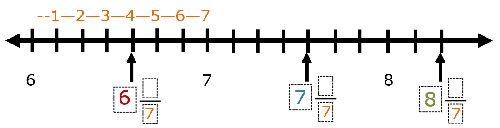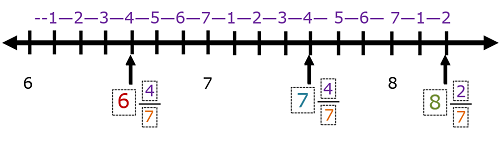# Math Worksheets Land

Math Worksheets For All Ages

# Math Worksheets Land

Math Worksheets For All Ages

# Mixed Numbers on a Number Line Worksheets

Mixed numbers are values that are composed of an integer and a proper. So mixed number values are more or less, depending on your viewpoint, than a whole unit of something. When you first see these values, they seem odd, but they are found in everyday life more than you think. They are found in all types of measurement calculations. When you are making a cake or any recipe for that matter you may need 2 1/2 cup of flour. I just had to measure my windows to determine the size curtain that I need and they had a width 3 1/4 feet. To help students better understand the concept of fractions we previously worked on displaying fractions on a number line. This just takes that skill a step further. We show students how to locate the whole number values and then further break down those intervals into more sections to allow them to represent the proper fractions. These worksheets and lessons will help you master this skill quickly and effectively.

### Aligned Standard: Grade 3 Fractions - 3.NF.2

• Answer Keys - These are for all the unlocked materials above.

### Practice Worksheets

Students make sure to take time to clearly understand what they are asking of you here.

• Practice 1 - Find the value of all the X points below. This means that you will need to write the mixed number out.
• Practice 2 - Fill in the missing whole numbers, mixed numbers, and proper fractions along the number line.
• Practice 3 - Find the position each point listed. This prepares us to read rulers that work off of the same principle.

### Homework Worksheets

We start stepping up the skill a bit. We remove more reference points to use as keys.

• HW 1 - Label the position by using the letter for each value. Note that each interval set is a different scale.
• HW 2 - We lead the students a bit because a single box indicates a whole number value.
• Skill Walkthrough - We show students how to master this skill in a full walk through.

### Quizzes

See how well you get the hang of this material. The quizzes are progressively more difficult.

• Quiz 1 - We slowly take away more reference points for students to reflect on.
• Quiz 2 - You are only given a single reference to base all your answers off of. Remember to label your way through it.

### How to Represent a Mixed Number on a Numbers Line

When we begin to use number lines we focus on the location of single whole numbers. A number line is a tool that helps kids learn to skip counting, addition, as well as subtraction. After a kid develops a strong understanding of locating a whole number on a number line, they progress to locating fractions and eventually mixed numbers on this same scale. This is where we begin to teach students that an interval can be broken into pieces, and this creates fractions and decimals. They are not normally used to represent percentages.

A mixed number is a number that consists of a whole number and a proper fraction. Locating proper fractions on a number line is easy, but a lot of kids find it difficult to locate mixed numbers on a number line. I encourage students to focus on locating the integer first and get that out of the way. Once you spot that whole number you have an idea as to which interval the fraction is located. You will just count to the correct interval from there.

Let us take a mixed number 2 4/5;

It consists of an integer, 2 and a proper fraction, 4/5. We can say that this mixed number will come between 2 and 3 on the number line as it is greater than 2 and smaller than 3. You start by dividing the segment between 2 and 3 in 5 equal parts. You would then locate the 4th segment in that series. That is the location on the numbers line.

### Take a Look at an Example Problem

Place the missing values on the numbers line.Step 1) Whole Number Values - Our first task is to fill in the missing whole number values. We can use the set whole numbers as a guide. As you will see below. Wherever that whole number value starts, it continues until the next whole number. You will see that we use a distinct color for each whole number value.Step 2) Find denominator of fraction - We count the number of intervals between each whole number. That value is the denominator of the fraction.Step 3) Find numerator of fraction - We count the number of intervals between each whole number and the point that the arrow lands at indicates the numerator of each fractional portion of the mixed number.Unlock all the answers, worksheets, homework, tests and more!
Save Tons of Time! Make My Life Easier Now

## Thanks and Don't Forget To Tell Your Friends!

I would appreciate everyone letting me know if you find any errors. I'm getting a little older these days and my eyes are going. Please contact me, to let me know. I'll fix it ASAP.